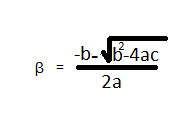# Quadratic Equations - How to Solve QuicklyToday I am sharing quick technique to solve Quadratic Equations quickly. I will also share practice questions on same to;

A polynomial of degree 2 is called Quadratic Equation.

For example:
• 2x2-5x+1=0
• x2-5=0

A general form of quadratic equation is ax2+bx+c=0

Where a, b, c all belong to real numbers

Now if you compare the equations I and II with the general form.
• a=2, b=-5 and c=1
• a=1, b=0 and c=-5

### Zeros or the solutions of Quadratic Equation

The real value of x for which the value of the P(x) = ax2+bx+c becomes zero is known as the root of

• Determine whether 3and 4 are the zeros of the polynomial P(x) =x2-7x+12
Solution:
P (3)   = 32-7.3+12
= 9-21+12
= -12+12
= 0

P (4) = 42-7.4+12
= 16-28+12
= -12+12
= 0
Therefore 3 and 4 are the zeros or the solutions or the roots of the polynomial P(x)=x2-7x+12

### Kinds of a Quadratic Equation

There are two types of quadratic equations

An equation of the form ax2+c=0 , a ≠0 is known as the pure quadratic equation .It means that the

quadratic equation ax2+bx+c =0, having no term containing single power of x, known as pure

quadratic equation . Clearly in a quadratic equation a ≠0 and b=0.

For example
• x2-4=0
• 3/8x2=5

A quadratic equation of the form ax2+bx+c =0, a ≠0 is known adfected quadratic equation or general

ax2+bx+c =0, a ≠0, b ≠0

For example:
• x2-5x+11=0
• 0.3x2+17x-2.3=0

## Solving a pure quadratic equation

There are two methods for solving a pure quadratic equation of the form ax2+c=0:
• By square root
• By factorization

### To solve a quadratic equation by square root

ax2+c=0 is a pure quadratic equation. To solve it , bring the constant term the RHS (right hand side)

and divide both side by a, coefficient of x2 and take the square root.

For example :

4x2-49=0

4x2=49

x2=49/4

x = +7/2

### To solve a pure quadratic equation by factorization

Bring the equation ax2+c=0 in the form p2-q2 =0. Use the p2-q2=(p+q)(p-q). Equate each factor to

zero  and find the values of x in each case, the two values of x so obtained are roots of the equation

ax2+c=0

For example :

16x2-25=0

(4x)2-(5)2 = 0

(4x+5) (4x-5) =0

4x-5 =0

x= 5/4

4x+5 =0

x =-5/4

There are two methods for solving an adfected quadratic equation ax2 + bx+c=0, a ≠0, b ≠0.

(i)                  By factorization                                         (ii)           By completing square

### To solve the quadratic equation ax2 + bx+c=0 by the method factorization

In this method the middle term (i.e term containing single power of x) is broken into two suitable

parts so that the factors are formed.

Example:             solve.    8x2 + 7x – 15 = 0

Solution:                                 8x2 +7x- 15 =0

Or                           8x2-8x + 15x-15=0

Or                           8x (x-1) +15(x-1) =0

Or                           (8x +15)(x-1)=0

Therefore                                      8x+15=0

8x=-15

x= -15/8
And                        x-1 =0

x-1
Hence   -15/8 and 1 are the required roots.

### To solve ax2 + bx+ c=0 by completion of  square

The famous Indian mathematician ShreedharAcharya had invented a formula for solving the

If the equation ax2 +bx +c =0 has roots α and β, thenandWhere      a = coefficient of x2

b = coefficient of x,

c = constant term